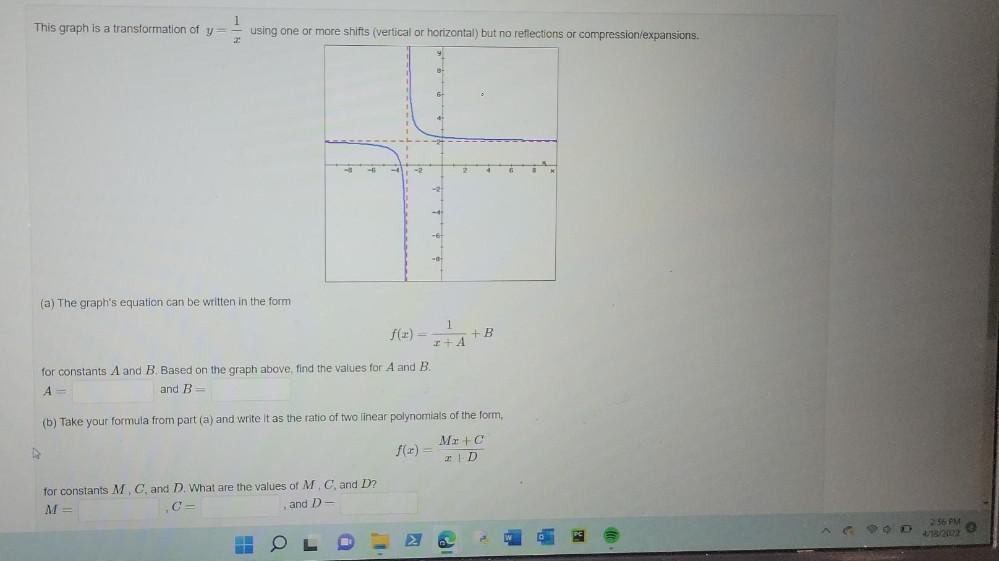Question:

# 1 This graph is a transformation of y= using one or more shifts (vertical or horizontal) but no reflections or compression/expan1 This graph is a transformation of y= using one or more shifts (vertical or horizontal) but no reflections or compression/expansions. 8 6 41 -6 2 6 8 (a) The graph's equation can be written in the form 1 f(x) = HAB + + for constants A and B. Based on the graph above, find the values for A and B. A= and B= (b) Take your formula from part (a) and write it as the ratio of two linear polynomials of the form, ho Mz+C f(2)= 2 +D for constants M, C, and D. What are the values of M. C, and D? M= C= and De 258 PM 4/18/2022